# Electrostatics

ElectromagnetismElectricity ·Magnetism
Electrostatics
Coulomb’s law · Electric flux · Gauss’ law · Electric potential · Electrostatic induction · Electric dipole moment ·

Electrostatics is a branch of science that involves the investigation of phenomena related to what appear to be stationary electric charges. Such phenomena are known as electrostatic phenomena.

It has been known since ancient times that the rubbing of some materials gives them the ability to attract small, light objects. Such materials were found to have acquired an electric charge. Examples of materials that can acquire a significant charge when rubbed together include amber rubbed with wool, glass rubbed with silk, and hard rubber rubbed with fur. This property, first recorded by Thales of Miletus, suggested the word "electricity" from the Greek word for amber, èlectròn.

Although electrostatically induced forces seem to be rather weak, the electrostatic force between an electron (which is negatively charged) and a proton (which is positively charged), together forming a hydrogen atom, is about 40 orders of magnitude stronger than the gravitational force acting between them.

The principles of electrostatics play an essential role in a number of technologies, including batteries, fuel cells, electroplating, thermocouples, radio detector diodes, photocells, light-emitting diodes (LEDs), thermoelectric cells, air filters, and xerography. However, undesirable charge accumulation has led to the damage of electronic components during manufacture, and the apparently spontaneous explosion of grain silos.

## History

The natural phenomenon of static electricity has been known since antiquity. It was recorded by Thales of Miletus in the sixth century B.C.E. Scientific research into the subject began when machines were built to create static electricity artificially, such as the friction generator developed by Otto von Guericke in the seventeenth century. The connection between static electricity and storm clouds was famously demonstrated by Benjamin Franklin in 1750.

In the late eighteenth century, scientists developed sensitive instruments for detecting "electrification," otherwise known as electrostatic charge imbalance. The phenomenon of electrification by contact, also known as contact electrification or contact tension, was quickly discovered. When two objects were touched together, sometimes the objects became spontaneously charged. One object developed a net negative charge, while the other developed an equal and opposite positive charge.

Based on the observation of contact electrification, scientists attempted to explain how electricity is generated in an electric battery, or the Voltaic pile, as it was then called. This theoretical view was eventually replaced by the current theory of electrochemistry, namely, that electricity is generated by the action of chemistry and the exchange of electrons between atoms making up the battery. An important fact leading to rejection of the theory of contact tension was the observation that corrosion (chemical degradation of the battery) seemed unavoidable with its use, and that the more electricity was drawn from the battery, the faster the corrosion proceeded.

The Volta effect does indeed correspond to a weak electric potential developed by the contact of different metals. This effect was first discovered by Alessandro Volta, and can be measured using a capacitance electroscope comprised of different metals. However, the actual effect is not sufficiently strong to account for the action of electric batteries.

A number of high voltage dry piles were invented between the early 1800s and the 1830s, in an attempt to explain static electricity, and specifically to support Volta’s hypothesis of contact tension. The Oxford Electric Bell is one example.

Until 1832, physicists thought that static electricity was somehow different from other forms of electricity. Then in 1832, Michael Faraday published the results of an experiment demonstrating that the electricity induced by a magnet, voltaic electricity produced by a battery, and static electricity were all the same. Since the time of Faraday's result, the history of static electricity merged with the study of electricity in general.

## Generation of static electricity

Static electricity is the buildup of electric charge on the surface of objects when they come in contact with each other and are then pulled apart. One surface acquires a positive charge, the other, a negative charge. Friction (rubbing of the surfaces) is not required, although in many situations it greatly enhances the phenomenon. Electrostatics involves study of the properties associated with such charge buildup.

Although charge exchange happens whenever any two surfaces come in contact and separate, the effects of charge exchange are usually noticed only when at least one of the surfaces has a high resistance to electrical flow. This is because the charges that transfer to or from the highly resistive surface are more or less trapped there for a long enough time for their effects to be observed. These charges then remain on the object until they either bleed off to ground or are quickly neutralized by a discharge.

For example, electric charge is built up when wool is rubbed against plastic or the soles of shoes rub on carpet. The mild shock one receives when touching a grounded object after walking on carpet is an example of excess electrical charge accumulating in one's body from frictional charging between one's shoes and the carpet. The resultant charge buildup within the body can generate a strong electrical discharge. Lightning is an example of giant electrical discharge in the atmosphere.

The process of how charges are built up on surfaces that come in contact with each other is not well understood. It may be caused by electron-stealing via quantum tunneling, or by transfer of surface ions. When negatively charged material touches a positively charged conductor, a spark is produced or a static shock is felt. A similar phenomenon occurs when a material that is positively charged touches a negatively charged conductor.

### Static electricity and chemical industry

Although experimenting with static electricity may be fun, sparks produced during electric discharge can create severe hazards in chemical industries that deal with flammable substances. In such cases, a small electrical spark may ignite explosive mixtures with devastating consequences.

A similar charging mechanism can occur within low-conductivity fluids flowing through pipelines—a process called flow electrification. Fluids that have low electrical conductivity (below 50 pico siemens/cm, where pico siemens/cm is a measure of electrical conductivity), are called accumulators. Fluids having conductivities above 50 pico siemens/cm are called non-accumulators. In non-accumulators, charges recombine as fast as they are separated and hence electrostatic charge generation is not significant. In the petrochemical industry, 50 pico siemens/cm is the recommended minimum value of electrical conductivity for adequate removal of charge from a fluid.

An important concept for insulating fluids is the static relaxation time. This is similar to the time constant (tau) within an RC circuit. For insulating materials, it is the ratio of the static dielectric constant divided by the electrical conductivity of the material. For hydrocarbon fluids, this is sometimes approximated by dividing the number 18 by the electrical conductivity of the fluid. Thus a fluid that has an electrical conductivity of one pico siemens /cm will have an estimated relaxation time of about 18 seconds. The excess charge within a fluid will be almost completely dissipated after four to five times the relaxation time, or 90 seconds for the fluid in the above example.

Charge generation increases at higher fluid velocities and larger pipe diameters, becoming quite significant in pipes 8 inches (200 mm) or larger. Static charge generation in these systems is best controlled by limiting fluid velocity.

Bonding and earthing are the usual ways by which charge buildup can be prevented. For fluids with electrical conductivity below ten pico siemens/cm, bonding and earthing are not adequate for charge dissipation, and anti-static additives may be required.

## Electrostatic generators

Usually insulators, that is, substances that do not conduct electricity, are good at both generating and holding a surface charge. Some examples of these substances are rubber, plastic, glass, and pith. Conductive objects only rarely generate charge imbalance except, for example, when a metal surface is impacted by solid or liquid nonconductors. The charge that is transferred during contact electrification is stored on the surface of each object. Static electric generators, devices which produce very high voltage at very low current and used for classroom physics demonstrations, rely on this effect.

## Charge neutralization

Natural electrostatic phenomena are most familiar as an occasional annoyance in seasons of low humidity, but they can be destructive and harmful in some situations (e.g. electronics manufacturing). When working in direct contact with integrated circuit electronics (especially delicate MOSFETs), or in the presence of flammable gas, care must be taken to avoid accumulating and suddenly discharging a static charge. To protect sensitive electronic components, manufacturers may use a number of antistatic devices.

## Charge induction

Charge induction occurs when a negatively charged object repels electrons from the surface of a second object. This creates a region in the second object that is more positively charged. An attractive force is then exerted between the objects. For example, when a balloon is rubbed, the balloon will stick to the wall as an attractive force is exerted by two oppositely charged surfaces (the surface of the wall gains an electric charge due to charge induction, as the free electrons at the surface of the wall are repelled by the negative balloon, creating a positive wall surface, which is subsequently attracted to the surface of the balloon). One can explore the effect with a simulation of the balloon and static electricity.

## Applications

Studies in the field of electrostatics have led to the development of a variety of modern electrical and electronic technologies such as batteries, fuel cells, electroplating, thermocouples, and semiconductor junction devices including radio detector diodes, photocells, light-emitting diodes (LEDs), and thermoelectric cells. In addition, the principles of electrostatics are commonly applied in xerography, air filters, and some automotive paints.

## Mathematical foundations

### Coulomb's law

The fundamental equation of electrostatics is Coulomb's law, which describes the force between two point charges. The magnitude of the electrostatic force between two point electric charges is directly proportional to the product of the magnitudes of each charge and inversely proportional to the square of the distance between the charges,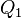$Q_1$ and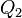$Q_2$:$F = \frac{Q_1Q_2}{4\pi\varepsilon_0 r^2}\ ,$

where ε0 is the electric constant, a defined value: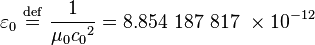$\varepsilon_0 \ \stackrel{\mathrm{def}}{=}\ \frac {1}{\mu_0 {c_0}^2} = 8.854\ 187\ 817\ \times 10^{-12}$   in A2s4 kg-1m−3 or C2N−1m−2 or F m−1.

### The electric field

The electric field (in units of volts per meter) is defined as the force (in newtons) per unit charge (in coulombs). From this definition and Coulomb's law, it follows that the magnitude of the electric field E created by a single point charge Q is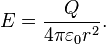$E = \frac{Q}{4\pi\varepsilon_0 r^2}.$

### Gauss's law

Gauss' law states that "the total electric flux through a closed surface is proportional to the total electric charge enclosed within the surface." The constant of proportionality is the permittivity of free space.

Mathematically, Gauss's law takes the form of an integral equation: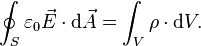$\oint_S\varepsilon_0\vec{E} \cdot\mathrm{d}\vec{A} = \int_V\rho\cdot\mathrm{d}V.$

Alternatively, in differential form, the equation becomes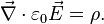$\vec{\nabla}\cdot\varepsilon_0\vec{E} = \rho.$

### The electrostatic approximation

The validity of the electrostatic approximation rests on the assumption that the electric field is irrotational: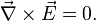$\vec{\nabla}\times\vec{E} = 0.$

From Faraday's law, this assumption implies the absence or near-absence of time-varying magnetic fields: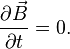${\partial\vec{B}\over\partial t} = 0.$

In other words, electrostatics does not require the absence of magnetic fields or electric currents. Rather, if magnetic fields or electric currents do exist, they must not change with time, or in the worst-case, they must change with time only very slowly. In some problems, both electrostatics and magnetostatics may be required for accurate predictions, but the coupling between the two can still be ignored.

### Electrostatic potential (voltage)

Because the electric field is irrotational, it is possible to express the electric field as the gradient of a scalar function, called the electrostatic potential (also known as the voltage). An electric field,$E$, points from regions of high potential, φ, to regions of low potential, expressed mathematically as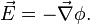$\vec{E} = -\vec{\nabla}\phi.$

### Poisson's equation

The definition of electrostatic potential, combined with the differential form of Gauss's law (above), provides a relationship between the potential φ and the charge density ρ: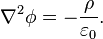${\nabla}^2 \phi = - {\rho\over\varepsilon_0}.$

This relationship is a form of Poisson's equation. Where${\varepsilon_0}$ is Vacuum permittivity.

### Laplace's equation

In the absence of unpaired electric charge, the equation becomes${\nabla}^2 \phi = 0,$

which is Laplace's equation.